# Defect(2)

(diff) ← Older revision | Latest revision (diff) | Newer revision → (diff)

ramification deficiency

An invariant of finite extensionsof fields equipped with a valuation (cf. also Extension of a field). If a valuation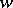onis the unique extension of a valuationon, then the defect (or ramification deficiency)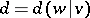is defined by the formula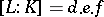, where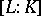is the degree of(i.e., the dimension ofas a-vector space),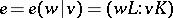is the ramification index and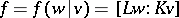is the inertia degree. Here,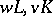denote the respective value groups and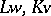the respective residue fields. Ifadmits several extensions to, the defectcan be defined by, whereis the number of distinct extensions, provided that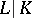is normal (since in that case the ramification index and the inertia degree are the same for all extensions; cf. also Normal extension).

In the above cases,,,are divisors of. The defectis either equal toor is a power of the characteristicof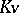if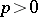; otherwise, it is always equal to(this is the Ostrowski lemma, cf. Ramification theory of valued fields).

## Henselian defect.

To avoid considering several valuations and to have a defect available for arbitrary finite extensions, one can pass to a Henselization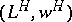ofand a Henselization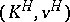of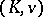inside(cf. Henselization of a valued field). The Henselian defect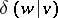is then defined to be the defect of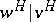(by the definition of the Henselization,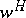is the unique extension of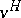). In the above cases,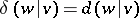.

## Defectless fields.

A fieldwith a valuationis called a defectless field if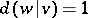for every finite normal extension. This holds if and only if the Henselian defect is equal tofor every finite extension. It follows thatis a defectless field if and only some Henselization ofis (or equivalently, all Henselizations are).

It follows from the Ostrowski lemma that all valued fields with residue field of characteristicare defectless fields. Also, valued fields of characteristicwith value group isomorphic to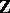are defectless. Combining both facts, it is shown that finitely ramified fields, and hence also fields with-valuations (see-adically closed field), are defectless.

If a valued field does not admit any non-trivial immediate extension (cf. also Valuation), then it is called a maximal valued field. Fields of formal Laurent series with their canonical valuations are maximal. Every maximal valued field is defectless.

## Fundamental inequality.

If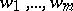are all extensions offromto, then one has the fundamental inequality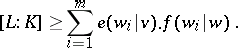This is an equality for every finiteif and only ifis defectless. Also, in general it can be written as an equality. For this, choose Henselizations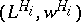of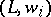andofinside. It is known that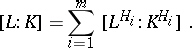Further,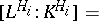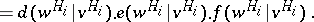Since Henselizations are immediate extensions,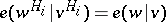and. By definition,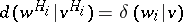. Hence,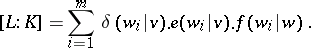Several other notions of defects were introduced. For a detailed theory of the defect, see [a1]. See also Valued function field.

How to Cite This Entry:
Defect(2). Encyclopedia of Mathematics. URL: http://encyclopediaofmath.org/index.php?title=Defect(2)&oldid=17106
This article was adapted from an original article by F.-V. Kuhlmann (originator), which appeared in Encyclopedia of Mathematics - ISBN 1402006098. See original article